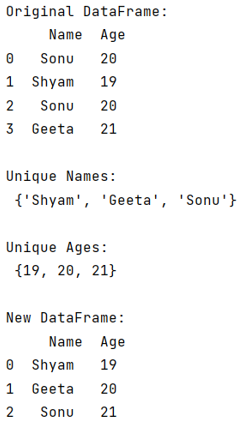# Python - Set difference for pandas

Learn, how to drop the duplicates and create a set difference for pandas dataframe? By Pranit Sharma Last updated : September 26, 2023

Pandas is a special tool that allows us to perform complex manipulations of data effectively and efficiently. Inside pandas, we mostly deal with a dataset in the form of DataFrame. DataFrames are 2-dimensional data structures in pandas. DataFrames consist of rows, columns, and data.

Sets are used to store multiple items which are heterogeneous. Just like list, tuple, and dictionary, the set is another built-in data type in python which is used to store elements. Elements inside a set are unique that is there is only 1 occurrence of each element inside a set.

It is believed that if we want to remove duplicates from any collection, the best way is to convert it into a set, but the set is a collection that is unordered, unchangeable*, and unindexed elements.

## Set difference for pandas

To drop the duplicates and create a set difference for the pandas dataframe, we will access all the columns and their values and map them into a variable which we will convert into a set.

Let us understand with the help of an example,

## Python program for set difference for pandas

```# Importing pandas package
import pandas as pd

# Creating a dictionary
d = {
'Name':["Sonu","Shyam","Sonu","Geeta"],
'Age':[20,19,20,21]
}

# Creating a DataFrame
df = pd.DataFrame(d)

# Display original DataFrame
print("Original DataFrame:\n",df,"\n")

# Accessing all the columns and converting
# them into set
set1 = set(df['Name'].values)
set2 = set(df['Age'].values)

# Display result
print("Unique Names:\n",set1,"\n")

print("Unique Ages:\n",set2,"\n")

# Creating DataFrame with these sets

set1 = list(set1)
set2 = list(set2)

df2 = pd.DataFrame({'Name':set1,'Age':set2})

# Print new dataframe
print("New DataFrame:\n",df2)
```

### Output

The output of the above program is: